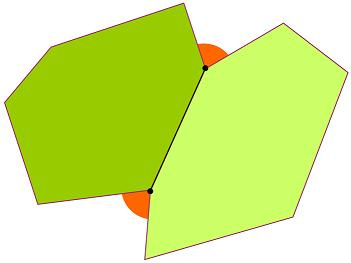#### You may also like### Some(?) of the Parts

A circle touches the lines OA, OB and AB where OA and OB are perpendicular. Show that the diameter of the circle is equal to the perimeter of the triangle### Polycircles

Show that for any triangle it is always possible to construct 3 touching circles with centres at the vertices. Is it possible to construct touching circles centred at the vertices of any polygon?### Circumspection

M is any point on the line AB. Squares of side length AM and MB are constructed and their circumcircles intersect at P (and M). Prove that the lines AD and BE produced pass through P.

# Two Regular Polygons

##### Age 14 to 16Challenge Level
Two polygons fit together so that the exterior (orange) angle at each end of their shared side is $81^\circ$.If both shapes now have to be regular polygons, but do not need to be the same, and each polygon can have any number of sides, could the orange angle still be $81^\circ$, and if that is possible how many sides would each polygon have?
Find solutions for when the orange angle is $27^\circ$ and when it is $54^\circ$.

Can you make a conjecture about the connection between the size of the orange angle and the number of sides on each polygon.
If you can, are you able to justify your conjecture?